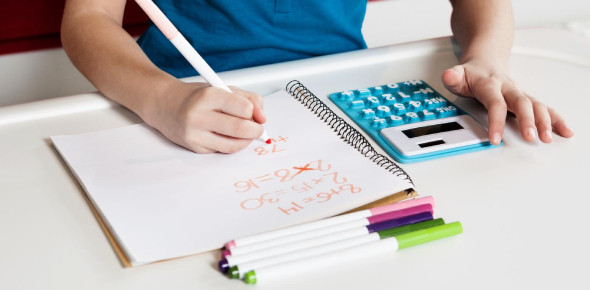# Use This As The First Maths Quiz For Your 1st Grade Child

20 Questions | Attempts: 96SettingsMathematics is one the fundamental and most important subject in a student's life. This quiz is designed for 1st grade math students who have just started studying maths, This quiz has some simple addition questions that will help your kid understand math better!

• 1.
4+5=
• 2.
8+6=
• 3.
2+9=
• 4.
5+8=
• 5.
8+1=
• 6.
7+3=
• 7.
7+10=
• 8.
8+2=
• 9.
9+4=
• 10.
9+6=
• 11.
5+6=
• 12.
2+5=
• 13.
7+5=
• 14.
3+5=
• 15.
8+8=
• 16.
4+4=
• 17.
3+10=
• 18.
8+7=
• 19.
2+3=
• 20.
9+7=

## Related TopicsBack to top
×

Wait!
Here's an interesting quiz for you.